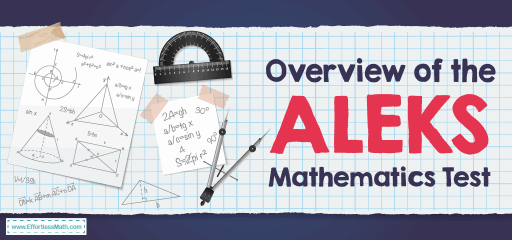# Overview of the ALEKS Mathematics TestThe Assessment and Learning in Knowledge Spaces (ALEKS) is an artificial-intelligence-based assessment tool that increases the weaknesses and strengths of students’ math knowledge, reports its findings to the student, and then a learning environment to enhance the student’s knowledge to the appropriate level for the placement. ALEKS, like most standardized tests, does not use multiple-choice questions. Instead, it uses an adaptable, easy-to-use method that mimics paper and pencil techniques.

The ALEKS Mathematics Assessment guarantees that students are prepared for particular math courses at the University of Oregon. Because these courses are demanding, students must start the course, which is likely to lead to their success. Students are not allowed to register in the math course unless they have demonstrated their readiness.

If a student is satisfied with his / her position after the initial assessment, he/she will be eligible to enroll in the appropriate course (s).  If the student is not satisfied with their placement after the initial assessment, they will be able to retake the assessment.

## What topics are covered on the ALEKS Mathematics Assessment?

ALEKS PPL is an online adaptation system that covers a wide range of math topics. The length of the placement assessment varies but can be up to 30 questions. Students may expect to see some, but not all, of the math, they may have learned in high school. ALEKS is a situational assessment, not a preview of math courses at the University of Oregon. It is designed to identify if you are ready for a particular course. After completing the initial placement assessment, students will have the opportunity to master additional topics to improve their placement.

### Mathematics topics include:

• Real numbers (including integers, fractions, and percentages)
• Equations and inequalities (including linear equations, systems of linear equations, linear inequalities, and quadratic equations)
• Quadratic and linear functions (including graphs and functions, linear functions, and parabolas)
• Exponents and polynomials (including integer exponents, factoring, polynomial arithmetic, and polynomial equations)
• Rational expressions (involve rational equations and rational functions)
• Radical expressions (including rational exponents and higher roots)
• logarithms and exponentials (involve properties of logarithms, function compositions and inverse functions, and logarithmic equations)
• Geometry and trigonometry (including area, perimeter, and volume, coordinate geometry, trigonometric functions, and identities and equations)

## Do you get a formula sheet on theALEKS Math Assessment?

The ALEKS Math test provides math formulas for some questions so you can focus on the application instead of memorizing the formulas. However, this test does not provide a list of all the mathematical formulas needed to know the test. It means that you should be able to recall many mathematical formulas in ALEKS.

## Is the ALEKS Math Assessment hard?

ALEKS Math Placement Assessment is just determining the level of math at a university to have a better chance of success. ALEKS Assessment measures your knowledge of mathematics up to pre-calculus. Therefore, it is difficult for many students to get a score above 75.

## Can you use a calculator on the ALEKSMath Test?

You don’t allow to use a personal calculator on the ALEKS Mathematics Assessment. But for some questions, the calculator button is active and the test taker can use it.

## How is the ALEKS Math Test scored?

ALEKS Mathematics Assessment score is between 1 and 100 and is interpreted as the correct percentage. A higher ALEKS score indicates that the examiner has mastered more mathematical concepts. The scores of 30 or higher show adequate preparation for university-level mathematics.

## More from Effortless Math for ALEKS Test …

### Want to know how to prepare for ALEKS math Test?

Check out our guide on How to Prepare for the ALEKS Math Test?

Practice test’s realistic format can help you succeed on the ALEKS Math test. Use our free ALEKS Math practice test and Full-Length ALEKS Math Practice Test to ace the ALEKS Math test!

### Need to know what is a good ALEKS score?

Have a look at What is a Good ALEKS Score?

## Have any questions about the ALEKS Test?

### What people say about "Overview of the ALEKS Mathematics Test - Effortless Math: We Help Students Learn to LOVE Mathematics"?

No one replied yet.

X
52% OFF

Limited time only!

Save Over 52%

SAVE \$40

It was \$76.99 now it is \$36.99